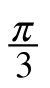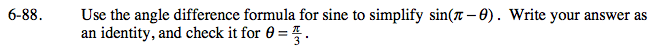### Home > PC > Chapter 6 > Lesson 6.2.2 > Problem6-88

6-88.

Use the angle difference formula for sine to simplify sin(π θ). Write your answer as an identity, and check it for θ =. Homework Help ✎sin(πθ) = sinπ cosθ − cosπ sinθ
= 0·cosθ − (−1) · sinθ
sin(π − θ) = sinθ